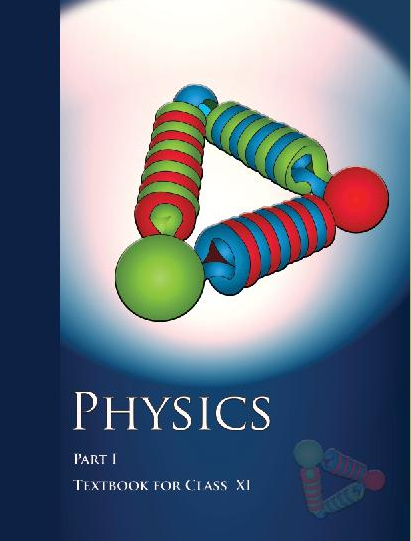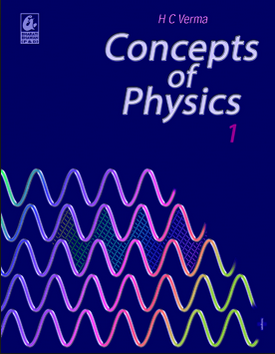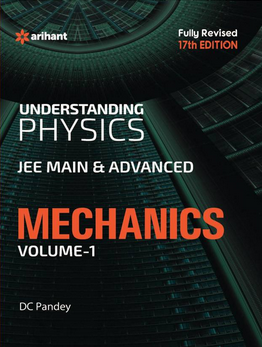# Work, energy and power (Weightage 6%)   Share

### Topics from Work, energy and power

• Work done by a constant force and variable force (12 concepts)
• conservative forces (3 concepts)
• Non - conservative forces (1 concepts)
• Conservation of mechanical energy ( kinetic and potential energies ) (3 concepts)
• Notion of potential energy (3 concepts)
• Kinetic energy (2 concepts)
• work-energy theorem (2 concepts)
• Work done by force (15 concepts)
• Kinetic and Potential Energy (6 concepts)
• Work Energy Theorem (2 concepts)
• Power (4 concepts)
• Potential energy of a spring (1 concepts)
• Conservation of energy (3 concepts)
• Elastic and Inelastic Collisions (11 concepts)

### Important Books for Work, energy and power

•••Exams
Articles
Questions